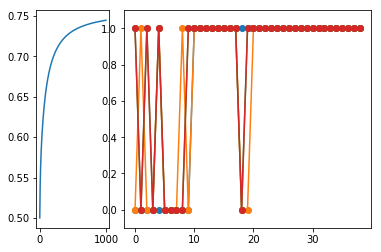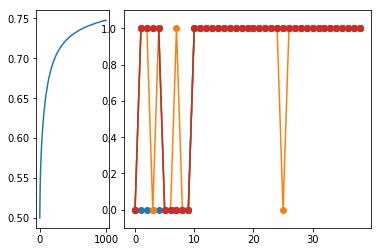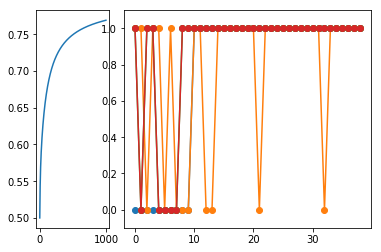$$\newcommand{\xv}{\mathbf{x}} \newcommand{\Xv}{\mathbf{X}} \newcommand{\yv}{\mathbf{y}} \newcommand{\Yv}{\mathbf{Y}} \newcommand{\zv}{\mathbf{z}} \newcommand{\av}{\mathbf{a}} \newcommand{\Wv}{\mathbf{W}} \newcommand{\wv}{\mathbf{w}} \newcommand{\betav}{\mathbf{\beta}} \newcommand{\gv}{\mathbf{g}} \newcommand{\Hv}{\mathbf{H}} \newcommand{\dv}{\mathbf{d}} \newcommand{\Vv}{\mathbf{V}} \newcommand{\vv}{\mathbf{v}} \newcommand{\tv}{\mathbf{t}} \newcommand{\Tv}{\mathbf{T}} \newcommand{\Sv}{\mathbf{S}} \newcommand{\zv}{\mathbf{z}} \newcommand{\Zv}{\mathbf{Z}} \newcommand{\Norm}{\mathcal{N}} \newcommand{\muv}{\boldsymbol{\mu}} \newcommand{\sigmav}{\boldsymbol{\sigma}} \newcommand{\phiv}{\boldsymbol{\phi}} \newcommand{\Phiv}{\boldsymbol{\Phi}} \newcommand{\Sigmav}{\boldsymbol{\Sigma}} \newcommand{\Lambdav}{\boldsymbol{\Lambda}} \newcommand{\half}{\frac{1}{2}} \newcommand{\argmax}{\underset{#1}{\operatorname{argmax}}} \newcommand{\argmin}{\underset{#1}{\operatorname{argmin}}} \newcommand{\dimensionbar}{\underset{#1}{\operatorname{|}}} \newcommand{\dimensionbar}{\underset{#1}{\operatorname{|}}} \newcommand{\grad}{\mathbf{\nabla}} \newcommand{\ebx}{e^{\wv_{#1}^T \xv_n}} \newcommand{\eby}{e^{y_{n,#1}}} \newcommand{\Tiv}{\mathbf{Ti}} \newcommand{\Fv}{\mathbf{F}} \newcommand{\ones}{\mathbf{1}_{#1}}$$

# Classification with Linear Logistic Regression¶

## Motivation and Setup¶

Recall that a linear model used for classification can result in masking. We discussed fixing this by using different shaped membership functions, other than linear.

Our first approach to this was to use generative models (Normal distributions) to model the data from each class, forming $p(\xv|C=k)$. Using Bayes Theorem, we converted this to $p(C=k|\xv)$ and derived QDA and LDA.

Now we will derive a linear model that directly predicts $p(C=k|\xv)$, resulting in the algorithm called logisitic regression. It is derived to maximize the likelihood of the data, given a bunch of samples and their class labels.

Remember this picture?The problem was that the green line for Class 2 was too low. In fact, all lines are too low in the middle of x range. Maybe we can reduce the masking effect by

• requiring the function values to be between 0 and 1, and
• requiring them to sum to 1 for every value of x.

We can satisfy those two requirements by directly representing $p(C=k|\xv)$ as

\begin{align*} p(C=k|\xv) = \frac{f(\xv;\wv_k)}{\sum_{m=1}^K f(\xv;\wv_m)} \end{align*}

with $f(\xv;\wv) \ge 0$. We haven't discussed the form of $f$ yet, but $\wv$ represents the parameters of $f$ that we will tune to fit the training data (later).

This is certainly an expression that is between 0 and 1 for any $\xv$. And we have $p(C=k|\xv)$ expressed directly, as opposed to the previous generative approach of first modeling $p(\xv|C=k)$ and using Bayes' theorem to get $p(C=k|\xv)$.

Let's give the above expression another name

\begin{align*} g_k(\xv) = p(C=k|\xv) = \frac{f(\xv;\wv_k)}{\sum_{m=1}^K f(\xv;\wv_m)} \end{align*}

## Derivation¶

Whatever we choose for $f$, we must make a plan for optimizing its parameters $\wv$. How?

Let's maximize the likelihood of the data. So, what is the likelihood of training data consisting of samples $\{\xv_1, \xv_2, \ldots, \xv_N\}$ and class indicator variables

\begin{align*} \begin{pmatrix} t_{1,1} & t_{1,2} & \ldots & t_{1,K}\\ t_{2,1} & t_{2,2} & \ldots & t_{2,K}\\ \vdots\\ t_{N,1} & t_{N,2} & \ldots & t_{N,K} \end{pmatrix} \end{align*}

with every value $t_{n,k}$ being 0 or 1, and each row of this matrix contains a single 1? (We can also express $\{\xv_1, \xv_2, \ldots, \xv_N\}$ as an $N \times D$ matrix, but we will be using single samples $\xv_n$ more often in the following.)

## Data Likelihood¶

The likelihood is just the product of all $p(C=\text{class of } n^\text{th}\text{ sample}\,|\,\xv_n)$ values for sample $n$. A common way to express this product, using those handy indicator variables is

\begin{align*} L(\betav) = \prod_{n=1}^N \prod_{k=1}^K p(C=k\,|\, \xv_n)^{t_{n,k}} \end{align*}

Say we have three classes ($K=3$) and training sample $n$ is from Class 2, then the product is

\begin{align*} p(C=1\,|\,\xv_n)^{t_{n,1}} p(C=2\,|\,\xv_n)^{t_{n,2}} p(C=3\,|\,\xv_n)^{t_{n,3}} & = p(C=1\,|\,\xv_n)^0 p(C=2\,|\,\xv_n)^1 p(C=3\,|\,\xv_n)^0 \\ & = 1\; p(C=2\,|\,\xv_n)^1 \; 1 \\ & = p(C=2\,|\,\xv_n) \end{align*}

This shows how the indicator variables as exponents select the correct terms to be included in the product.

## Maximizing the Data Likelihood¶

So, we want to find $\wv$ that maximizes the data likelihood. How shall we proceed?

\begin{align*} L(\wv) & = \prod_{n=1}^N \prod_{k=1}^K p(C=k\,|\, \xv_n) ^ {t_{n,k}} \end{align*}

Right. Find the derivative with respect to each component of $\wv$, or the gradient with respect to $\wv$. But there is a mess of products in this. So...

Right again. Work with the natural logarithm $\log L(\wv)$ which we will call $LL(\wv)$.

\begin{align*} LL(\wv) = \log L(\wv) = \sum_{n=1}^N \sum_{k=1}^K t_{n,k} \log p(C=k\,|\,\xv_n) \end{align*}

## Gradient Ascent¶

Unfortunately, the gradient of $LL(\wv)$ with respect to $\wv$ is not linear in $\wv$, so we cannot simply set the result equal to zero and solve for $\wv$.

Instead, we do gradient ascent. (Why "ascent"?)

• Initialize $\wv$ to some value.
• Make small change to $\wv$ in the direction of the gradient of $LL(\wv)$ with respect to $\wv$ (or $\grad_{\wv} LL(\wv)$)
• Repeat above step until $LL(\wv)$ seems to be at a maximum.
\begin{align*} \wv \leftarrow \wv + \alpha \grad_{\wv} LL(\wv) \end{align*}

where $\alpha$ is a constant that affects the step size.

Remember that $\wv$ is a matrix of parameters, with, let's say, columns corresponding to the values required for each $f$, of which there are $K-1$.

We can work on the update formula and $\grad_{\wv} LL(\wv)$ one column at a time

\begin{align*} \wv_k \leftarrow \wv_k + \alpha \grad_{\wv_k} LL(\wv) \end{align*}

and combine them at the end.

\begin{align*} \wv \leftarrow \wv + \alpha (\grad_{\wv_1} LL(\wv), \grad_{\wv_2} LL(\wv), \ldots, \grad_{\wv_{K-1}} LL(\wv)) \end{align*}

Remembering that $\frac{\partial \log h(x)}{\partial x} = \frac{1}{h(x)}\frac{\partial h(x)}{x}$ and that $p(C=k|\xv_n) = g_k(\xv_n)$

\begin{align*} LL(\wv) & = \sum_{n=1}^N \sum_{k=1}^K t_{n,k} \log p(C=k\,|\,\xv_n)\\ & = \sum_{n=1}^N \sum_{k=1}^K t_{n,k} \log g_k(\xv_n)\\ \grad_{\wv_j} LL(\wv) & = \sum_{n=1}^N \sum_{k=1}^K \frac{t_{n,k}}{g_k(\xv_n)} \grad_{\wv_j} g_k(\xv_n) \end{align*}

It would be super nice if $\grad_{\wv_j} g_k(\xv_n)$ includes the factor $g_k(\xv_n)$ so that it will cancel with the $g_k(\xv_n)$ in the denominator.

Can get this by defining

\begin{align*} f(\xv_n;\wv_k) & = \ebx{k} \;\;\;\;\text{ so}\\ g_k(\xv_n) & = \frac{f(\xv_n;\wv_k)}{\sum_{m=1}^{K} f(\xv_n;\wv_m)} \end{align*}

Now we can work on $\grad_{\wv_j} g_k(\xv_n)$.

\begin{align*} g_k(\xv_n) = \frac{\ebx{k}}{\sum_{m=1}^{K} \ebx{m}} \end{align*}

So

\begin{align*} \grad_{\wv_j} g_k(\xv_n) & = \grad_{\wv_j} \left (\frac{\ebx{k}}{\sum_{m=1}^{K} \ebx{m}} \right )\\ & = \grad_{\wv_j} \left [ \left (\sum_{m=1}^{K} \ebx{m} \right )^{-1} \ebx{k} \right ] \end{align*}

Since \begin{align*} \grad_{\wv_j} \ebx{k} &= \begin{cases} \xv_n \ebx{k}, & \text{if } k=j\\ 0 & \text{otherwise} \end{cases} \end{align*} and \begin{align*} \grad_{\wv_j} \sum_{m=1}^K-1 \ebx{m} &= \xv_n \ebx{k} \end{align*} then \begin{align*} \grad_{\wv_j} g_k(\xv_n) & = \grad_{\wv_j} \left (\frac{\ebx{k}}{\sum_{m=1}^{K} \ebx{m}} \right )\\ & = -1 \left (\sum_{m=1}^{K} \ebx{m} \right )^{-2} \xv_n \ebx{j} \ebx{k} + \left (\sum_{m=1}^{K} \ebx{m} \right )^{-1} \begin{cases} \xv_n \ebx{k},& \text{if} j=k\\ 0,& \text{otherwise} \end{cases}\\ & = -\frac{\ebx{k}}{\sum_{m=1}^{K} \ebx{m}} \frac{\ebx{j}}{\sum_{m=1}^{K} \ebx{j}} \xv_n + \begin{cases} \frac{\ebx{j}}{\sum_{m=1}^{K} \ebx{j}} \xv_n,& \text{if} j=k\\ 0,& \text{otherwise} \end{cases}\\ %& = \frac{\ebx{k}}{\sum_{m=1}^{K} \ebx{m} } & = - g_k(\xv_n) g_j(\xv_n) \xv_n + \begin{cases} g_j(\xv_n) \xv_n,^ \text{if} j=k\\ 0,& \text{otherwise} \end{cases}\\ & = g_k(\xv_n) (\delta_{jk} - g_j(\xv_n)) \xv_n \end{align*} where $\delta_{jk} = 1$ if $j=k$, 0 otherwise.

Substituting this back into the log likelihood expression, we get

\begin{align*} \grad_{\wv_j} LL(\wv) & = \sum_{n=1}^N \sum_{k=1}^K \frac{t_{n,k}}{g_k(\xv_n)} \grad_{\wv_j} g_k(\xv_n)\\ & = \sum_{n=1}^N \sum_{k=1}^K \frac{t_{n,k}}{g_k(\xv_n)} \left (g_k(\xv_n) (\delta_{jk} - g_j(\xv_n)) \xv_n \right )\\ & = \sum_{n=1}^N \left ( \sum_{k=1}^K t_{n,k} \delta_{jk} - g_j(\xv_n) \sum_{k=1}^K t_{n,k} \right ) \xv_n\\ & = \sum_{n=1}^N (t_{n,j} - g_j(\xv_n)) \xv_n \end{align*}

which results in this update rule for $\wv_j$

\begin{align*} \wv_j \leftarrow \wv_j + \alpha \sum_{n=1}^N (t_{n,j} - g_j(\xv_n)) \xv_n \end{align*}

How do we do this in python? First, a summary of the derivation.

## Derivation Summary¶

$P(C=k\,|\,\xv_n)$ and the data likelihood we want to maximize:

\begin{align*} g_k(\xv_n) & = P(C=k\,|\,\xv_n) = \frac{f(\xv_n;\wv_k)}{\sum_{m=1}^{K} f(\xv_n;\wv_m)}\\ f(\xv_n;\wv_k) & = \left \{ \begin{array}{ll} \ebx{k}; & k < K\\ 1;& k = K \end{array} \right .\\ L(\wv) & = \prod_{n=1}^N \prod_{k=1}^K p(C=k\,|\, \xv_n) ^{t_{n,k}}\\ & = \prod_{n=1}^N \prod_{k=1}^K g_k(\xv_n)^{t_{n,k}} \end{align*}

Gradient of log likelihood with respect to $\wv_j$:

\begin{align*} \grad_{\wv_j} LL(\wv) & = \sum_{n=1}^N \sum_{k=1}^K \frac{t_{n,k}}{g_k(\xv_n)} \grad_{\wv_j} g_k(\xv_n)\\ %& = \sum_{n=1}^N \left ( \sum_{k=1}^K t_{n,k} \delta_{jk} - % g_j(\xv_n) \sum_{k=1}^K t_{n,k} \right )\\ & = \sum_{n=1}^N \xv_n (t_{n,j} - g_j(\xv_n)) \end{align*}

which results in this update rule for $\wv_j$

\begin{align*} \wv_j \leftarrow \wv_j + \alpha \sum_{n=1}^N (t_{n,j} - g_j(\xv_n)) \xv_n \end{align*}

## Implementation in Python¶

Update rule for $\wv_j$

\begin{align*} \wv_j \leftarrow \wv_j + \alpha \sum_{n=1}^N (t_{n,j} - g_j(\xv_n)) \xv_n \end{align*}

What are shapes of each piece? Remember that whenever we are dealing with weighted sums of inputs, as we are here, add the constant 1 to the front of each sample.

• $\xv_n$ is $(D+1) \times 1$ ($+1$ for the constant 1 input)
• $\wv_j$ is $(D+1) \times 1$
• $t_{n,j} - g_j(\xv_n)$ is a scalar

So, this all works. But, notice the sum is over $n$, and each term in the product as $n$ components, so we can do this as a dot product.

Let's remove the sum and replace subscript $n$ with *.

\begin{align*} \wv_j &\leftarrow \wv_j + \alpha \sum_{n=1}^N (t_{n,j} - g_j(\xv_n)) \xv_n\\ \wv_j &\leftarrow \wv_j + \alpha (t_{*,j} - g_j(\xv_*)) \xv_*\\ \end{align*}

What are shapes of each piece?

• $(t_{*,j} - g_j(\xv_*))$ is $N \times 1$
• $\xv_* = X$ is $N \times (D+1)$
• $\wv_j$ is $(D+1) \times 1$

So, this will work if we transpose $X$ and premultiply it and define $g$ as a function that accepts $\Xv$.

\begin{align*} % \wv_j &\leftarrow \wv_j + \alpha (t_{*,j} - % g(\xv_*;\wv_j)) \xv_*\\ \wv_j &\leftarrow \wv_j + \alpha \Xv^T (t_{*,j} - g_j(\Xv)) \end{align*}

Let's keep going...and try to make this expression work for all of the $\wv$'s. Playing with the subscripts again, replace $j$ with *.

\begin{align*} \wv_j &\leftarrow \wv_j + \alpha \Xv^T (t_{*,j} - g_j(\Xv))\\ \wv_* &\leftarrow \wv_* + \alpha \Xv^T (t_{*,*} - g_*(\Xv)) \end{align*}

Now what are shapes?

• $\wv_* = \wv$ is $(D+1) \times K$
• $t_{*,*} = T$ is $N \times K$
• $g_*(\Xv)$ is $N \times (K-1)$
• $t_{*,*} - g_*(\Xv)$ is $N \times K$
• So, $\Xv^T (t_{*,*} - g_*(\Xv))$ is $(D+1) \times K$
• So, $\Xv^T (T - g(\Xv))$ is $(D+1) \times K$

Now our update equation for all $\wv$'s is

\begin{align*} \wv &\leftarrow \wv + \alpha \Xv^T (T - g(\Xv)) \end{align*}

We had defined, for $k = 1,\ldots, K$,

\begin{align*} f(\xv_n;\wv_k) & = \ebx{k} \\ g_k(\xv) &= \dfrac{f(\xv;\wv_k)}{\sum_{m=1}^K f(\xv;\wv_m)} \end{align*}

Changing these to handle all samples $\Xv$ and all parameters $\wv$ we have

\begin{align*} f(\Xv;\wv) & = e^{\Xv \wv}\\ g(\Xv) & = \frac{f(\Xv;\wv)}{\text{rowSums}(f(\Xv;\wv)} \end{align*}

Given training data $\Xv$ ($N\times (D+1)$) and class indicator variables $T$ ($N \times K)$), these expressions can be performed with the following code.

First, we need a function to create indicator variables from the class labels, to get

$$\begin{bmatrix} 1\\ 2\\ 2\\ 1\\ 3 \end{bmatrix} \Rightarrow \begin{bmatrix} 1 & 0 & 0\\ 0 & 1 & 0\\ 0 & 1 & 0\\ 1 & 0 & 0\\ 0 & 0 & 1 \end{bmatrix}$$
In :
import numpy as np
import matplotlib.pyplot as plt
%matplotlib inline

In :
def makeIndicatorVars(T):
# Make sure T is two-dimensiona. Should be nSamples x 1.
if T.ndim == 1:
T = T.reshape((-1,1))
return (T == np.unique(T)).astype(int)

In :
T = np.array([1,2,2,1,3]).reshape((-1,1))
T

Out:
array([,
,
,
,
])
In :
makeIndicatorVars(T)

Out:
array([[1, 0, 0],
[0, 1, 0],
[0, 1, 0],
[1, 0, 0],
[0, 0, 1]])
In :
# import pdb
def g(X,w):
fs = np.exp(np.dot(X, w))  # N x K
denom = np.sum(fs,axis=1).reshape((-1,1))
# pdb.set_trace()
gs = fs / denom
# print(gs[:10,:])
return gs


The function g is sometimes called the softmax function.

Now the updates to $\wv$ can be formed with code like

TI = makeIndicatorVars(T)
w = np.zeros((X.shape,TI.shape))
alpha = 0.0001
for step in range(1000):
gs = g(X,w)
# Error does not involve the last column of indicator variables in TI nor gs
w = w + alpha * np.dot(X.T, TI - gs)



Here is code for applying linear logistic regression to the Parkinsons data from last lecture.

In :
! curl -O https://archive.ics.uci.edu/ml/machine-learning-databases/parkinsons/parkinsons.data

  % Total    % Received % Xferd  Average Speed   Time    Time     Time  Current
Dload  Upload   Total   Spent    Left  Speed
100 40697  100 40697    0     0   160k      0 --:--:-- --:--:-- --:--:--  160k

In :
def loadParkinsonsData(filename='parkinsons.data'):
with open(filename,"r") as f:
header = f.readline()
names = header.strip().split(',')[1:]

data = np.loadtxt(f ,delimiter=',', usecols=1+np.arange(23))

targetColumn = names.index("status")
XColumns = np.arange(23)
XColumns = np.delete(XColumns, targetColumn)
X = data[:,XColumns]
T = data[:,targetColumn].reshape((-1,1)) # to keep 2-d matrix form
names.remove("status")
return X,T,names

In :
X,T,names = loadParkinsonsData()

In :
X.shape,T.shape,names

Out:
((195, 22),
(195, 1),
['MDVP:Fo(Hz)',
'MDVP:Fhi(Hz)',
'MDVP:Flo(Hz)',
'MDVP:Jitter(%)',
'MDVP:Jitter(Abs)',
'MDVP:RAP',
'MDVP:PPQ',
'Jitter:DDP',
'MDVP:Shimmer',
'MDVP:Shimmer(dB)',
'Shimmer:APQ3',
'Shimmer:APQ5',
'MDVP:APQ',
'Shimmer:DDA',
'NHR',
'HNR',
'RPDE',
'DFA',
'spread1',
'spread2',
'D2',
'PPE'])
In :
def standardize(X,mean,stds):
return (X - mean)/stds

In :
! curl -O http://www.cs.colostate.edu/~anderson/cs445/notebooks/qdalda.py

  % Total    % Received % Xferd  Average Speed   Time    Time     Time  Current
Dload  Upload   Total   Spent    Left  Speed
100   340  100   340    0     0   110k      0 --:--:-- --:--:-- --:--:--  110k

In :
import qdalda

In :
def runParkLogReg(filename, trainFraction):
f = open(filename,"r")
header = f.readline()
names = header.strip().split(',')[1:]

data = np.loadtxt(f ,delimiter=',', usecols=1+np.arange(23))

targetColumn = names.index("status")
XColumns = np.arange(23)
XColumns = np.delete(XColumns, targetColumn)
X = data[:, XColumns]
T = data[:, targetColumn].reshape((-1, 1)) # to keep 2-d matrix form
names.remove("status")

healthyI,_ = np.where(T == 0)
parkI,_ = np.where(T == 1)
healthyI = np.random.permutation(healthyI)
parkI = np.random.permutation(parkI)

nHealthy = round(trainFraction*len(healthyI))
nPark = round(trainFraction*len(parkI))
rowsTrain = np.hstack((healthyI[:nHealthy], parkI[:nPark]))
Xtrain = X[rowsTrain, :]
Ttrain = T[rowsTrain, :]
rowsTest = np.hstack((healthyI[nHealthy:], parkI[nPark:]))
Xtest =  X[rowsTest, :]
Ttest =  T[rowsTest, :]

means,stds = np.mean(Xtrain, 0), np.std(Xtrain ,0)
Xtrains = standardize(Xtrain, means, stds)
Xtests = standardize(Xtest, means, stds)

Xtrains1 = np.hstack(( np.ones((Xtrains.shape, 1)), Xtrains))
Xtests1 = np.hstack(( np.ones((Xtests.shape, 1)), Xtests))

# New stuff for linear logistic regression

TtrainI = makeIndicatorVars(Ttrain)
TtestI = makeIndicatorVars(Ttest)

w = np.zeros((Xtrains1.shape, TtrainI.shape))
likelihood = []
alpha = 0.0001
for step in range(1000):
gs = g(Xtrains1, w)
w = w + alpha * np.dot(Xtrains1.T, TtrainI - gs)
likelihoodPerSample = np.exp( np.sum(TtrainI * np.log(gs)) / Xtrains.shape)
likelihood.append(likelihoodPerSample)
# print("Step",step," l =",likelihoodPerSample)

plt.subplot2grid((1, 4), (0, 0))
plt.plot(likelihood)

logregOutput = g(Xtrains1, w)
predictedTrain = np.argmax(logregOutput, axis=1)
logregOutput = g(Xtests1, w)
predictedTestLR = np.argmax(logregOutput, axis=1)

print("LogReg: Percent correct: Train {:.3g} Test {:.3g}".format(percentCorrect(predictedTrain, Ttrain),
percentCorrect(predictedTestLR, Ttest)))

# Previous QDA, LDA code

qda = qdalda.QDA()
qda.train(Xtrain, Ttrain)
qdaPredictedTrain = qda.use(Xtrain)
qdaPredictedTest = qda.use(Xtest)
print("   QDA: Percent correct: Train {:.3g} Test {:.3g}".format(percentCorrect(qdaPredictedTrain, Ttrain),
percentCorrect(qdaPredictedTest, Ttest)))

lda = qdalda.LDA()
lda.train(Xtrain, Ttrain)
ldaPredictedTrain = qda.use(Xtrain)
ldaPredictedTest = qda.use(Xtest)
print("   LDA: Percent correct: Train {:.3g} Test {:.3g}".format(percentCorrect(ldaPredictedTrain, Ttrain),
percentCorrect(ldaPredictedTest, Ttest)))

plt.subplot2grid((1, 4), (0, 1), colspan=3)
plt.plot(Ttest, 'o-')
plt.plot(predictedTestLR, 'o-')
plt.plot(qdaPredictedTest, 'o-')
plt.plot(ldaPredictedTest, 'o-')
plt.ylim(-0.1, 1.1)

def percentCorrect(p, t):
return np.sum(p.ravel()==t.ravel()) / float(len(t)) * 100

def discQDA(X, means, stds, mu, Sigma, prior):
Xc = standardize(X, means, stds) - mu
if Sigma.size == 1:
Sigma = np.asarray(Sigma).reshape((1, 1))
det = np.linalg.det(Sigma)
if det == 0:
raise np.linalg.LinAlgError('discQDA(): Singular covariance matrix')
SigmaInv = np.linalg.inv(Sigma)     # pinv in case Sigma is singular
return -0.5 * np.log(det) \
- 0.5 * np.sum(np.dot(Xc, SigmaInv) * Xc, axis=1).reshape((-1,1)) \
+ np.log(prior)

In :
runParkLogReg('parkinsons.data',0.8)

LogReg: Percent correct: Train 85.3 Test 87.2
QDA: Percent correct: Train 98.1 Test 87.2
LDA: Percent correct: Train 98.1 Test 87.2In :
runParkLogReg('parkinsons.data',0.8)

LogReg: Percent correct: Train 85.9 Test 87.2
QDA: Percent correct: Train 98.1 Test 89.7
LDA: Percent correct: Train 98.1 Test 89.7In :
runParkLogReg('parkinsons.data',0.8)

LogReg: Percent correct: Train 87.8 Test 76.9
QDA: Percent correct: Train 99.4 Test 87.2
LDA: Percent correct: Train 99.4 Test 87.2The code above is doing steepest ascent in the gradient of the log likelihood. Do we have a better way of doing this gradient ascent?

Hey, how about using Moller's Scaled Conjugate Gradient again? Just have to define the function being optimized and its gradient. The function to be optimized should be the negative of the log likelihood, because SCG is designed to minimize the function. And the gradient function must also include this negative. But with these negatives, SCG will work fine for optimizing the weights in a linear logistic regression classifier.

This is left for you to do. You will get clues about how to do this from the neural network implementation of nonlinear logistic regression in the next set of notes.

Here are definitions of the log likehood and its gradient again. \begin{align*} LL(\wv) & = \sum_{n=1}^N \sum_{k=1}^K t_{n,k} \log g_k(\xv_n)\\ \grad_{\wv_j} LL(\wv) & = \sum_{n=1}^N \xv_n (t_{n,j} - g_j(\xv_n)) \end{align*}

or, as matrices, and using the mean log likelihood,

\begin{align*} Y &= g(\Xv)\\ LL(\wv) & = \text{np.mean}(T \cdot \log Y , \text{axis}=0) \\ \grad_{\wv_j} LL(\wv) & = \Xv^T (T - Y) \;/\; (N\,K) \end{align*}
In :
!curl -O http://www.cs.colostate.edu/~anderson/cs445/notebooks/mlutilities.tar
!tar xvf mlutilities.tar

  % Total    % Received % Xferd  Average Speed   Time    Time     Time  Current
Dload  Upload   Total   Spent    Left  Speed
100 30720  100 30720    0     0  14.6M      0 --:--:-- --:--:-- --:--:-- 14.6M
mlutilities.py

In :
import mlutilities as ml

def runParkLogReg2(filename, trainFraction):
f = open(filename,"r")
header = f.readline()
names = header.strip().split(',')[1:]

data = np.loadtxt(f ,delimiter=',', usecols=1+np.arange(23))

targetColumn = names.index("status")
XColumns = np.arange(23)
XColumns = np.delete(XColumns, targetColumn)
X = data[:,XColumns]
T = data[:,targetColumn].reshape((-1,1)) # to keep 2-d matrix form
names.remove("status")

healthyI,_ = np.where(T == 0)
parkI,_ = np.where(T == 1)
healthyI = np.random.permutation(healthyI)
parkI = np.random.permutation(parkI)

nHealthy = round(trainFraction*len(healthyI))
nPark = round(trainFraction*len(parkI))
rowsTrain = np.hstack((healthyI[:nHealthy], parkI[:nPark]))
Xtrain = X[rowsTrain,:]
Ttrain = T[rowsTrain,:]
rowsTest = np.hstack((healthyI[nHealthy:], parkI[nPark:]))
Xtest =  X[rowsTest,:]
Ttest =  T[rowsTest,:]

means,stds = np.mean(Xtrain,0), np.std(Xtrain,0)
Xtrains = standardize(Xtrain,means,stds)
Xtests = standardize(Xtest,means,stds)

Xtrains1 = np.hstack(( np.ones((Xtrains.shape,1)), Xtrains))
Xtests1 = np.hstack(( np.ones((Xtests.shape,1)), Xtests))

# New stuff for linear logistic regression

TtrainI = makeIndicatorVars(Ttrain)
TtestI = makeIndicatorVars(Ttest)
K = TtrainI.shape

def loglikelihood(warg,K):
w = warg.reshape((-1,K))
Y = g(Xtrains1,w)
# print(w)
return - np.mean(TtrainI*np.log(Y))

def gradientloglikelihood(warg,K):
w = warg.reshape((-1,K))
# print('w',w)
N = Xtrains1.shape
Y = g(Xtrains1,w)
# print('Y',Y[:10,:])
# print('Xtrains1',Xtrains1[:5,:])
# print('TtrainI',TtrainI[:5,:])
# print('dot',np.dot(Xtrains1.T,(Y-TtrainI)[:,:-1]))
# print('N',N,'K',K)
grad = np.dot(Xtrains1.T, Y-TtrainI) / (N * K)
return grad.reshape((-1))

w = np.zeros((Xtrains1.shape,TtrainI.shape))
resultSCG = ml.scg(w.reshape((-1)), loglikelihood, gradientloglikelihood, K, nIterations=100, ftracep=True, verbose=False)
wresult = resultSCG['x']
w = wresult.reshape((-1,K))

logregOutput = g(Xtrains1,w)
predictedTrain = np.argmax(logregOutput,axis=1)
logregOutput = g(Xtests1,w)
predictedTest = np.argmax(logregOutput,axis=1)

print("LogReg SCG: Percent correct: Train {:.3g} Test {:.3g}".format(percentCorrect(predictedTrain,Ttrain),percentCorrect(predictedTest,Ttest)))

# Previous QDA code

qda = qdalda.QDA()
qda.train(Xtrain, Ttrain)
qdaPredictedTrain = qda.use(Xtrain)
qdaPredictedTest = qda.use(Xtest)
print("   QDA: Percent correct: Train {:.3g} Test {:.3g}".format(percentCorrect(qdaPredictedTrain, Ttrain),
percentCorrect(qdaPredictedTest, Ttest)))

lda = qdalda.LDA()
lda.train(Xtrain, Ttrain)
ldaPredictedTrain = qda.use(Xtrain)
ldaPredictedTest = qda.use(Xtest)
print("   LDA: Percent correct: Train {:.3g} Test {:.3g}".format(percentCorrect(ldaPredictedTrain, Ttrain),
percentCorrect(ldaPredictedTest, Ttest)))

In :
runParkLogReg2('parkinsons.data',0.8)

LogReg SCG: Percent correct: Train 90.4 Test 84.6
QDA: Percent correct: Train 97.4 Test 87.2
LDA: Percent correct: Train 97.4 Test 87.2

In :
runParkLogReg2('parkinsons.data',0.8)

LogReg SCG: Percent correct: Train 88.5 Test 94.9
QDA: Percent correct: Train 98.7 Test 84.6
LDA: Percent correct: Train 98.7 Test 84.6

In [ ]: